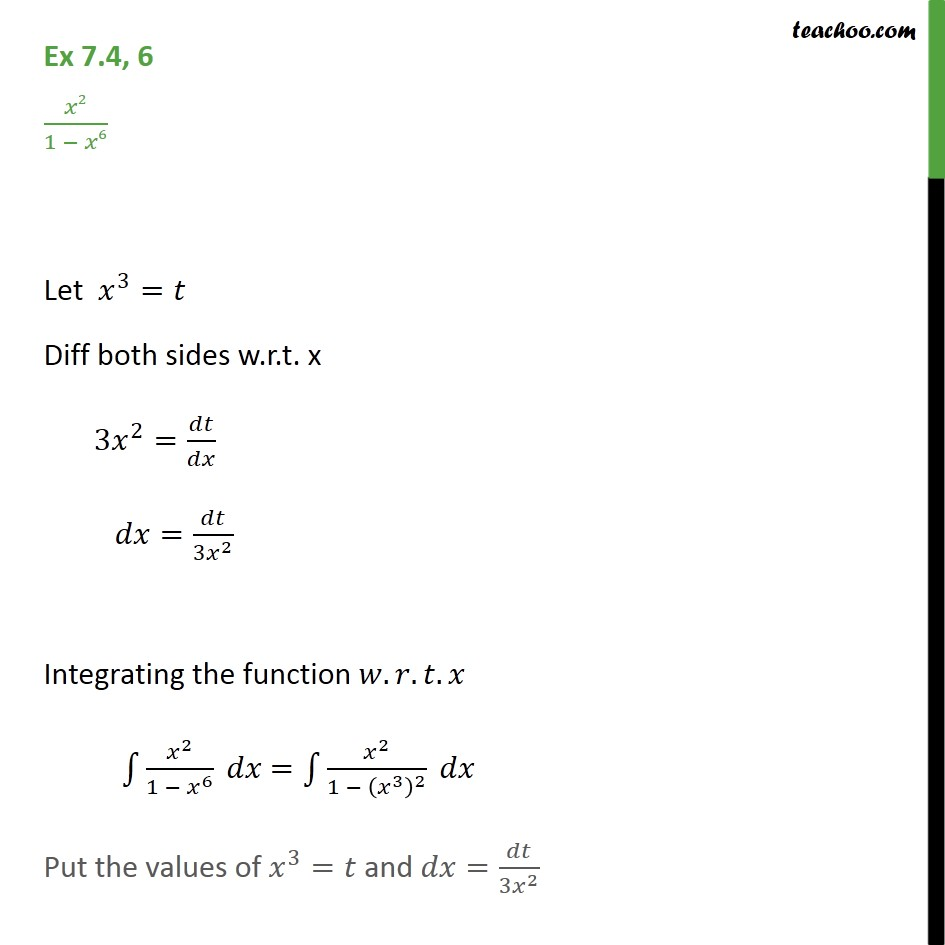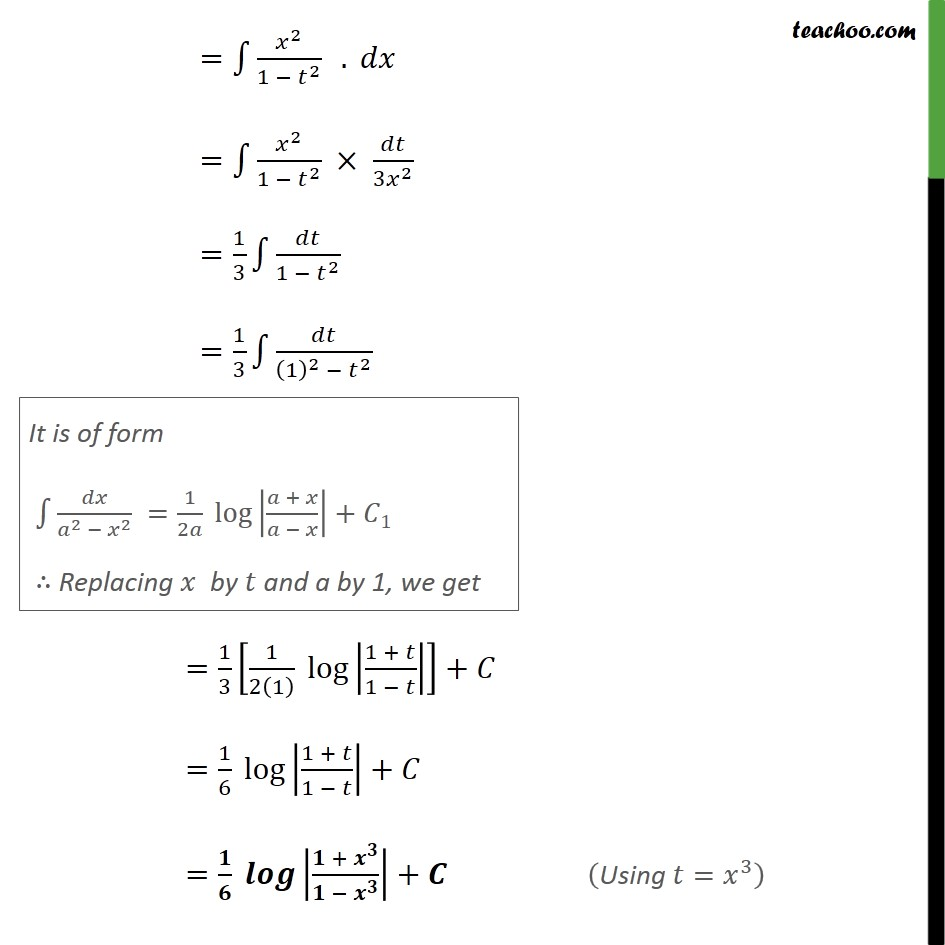Ex 7.4

Chapter 7 Class 12 Integrals
Serial order wiseLearn in your speed, with individual attention - Teachoo Maths 1-on-1 Class

### Transcript

Ex 7.4, 6 2/(1 6) Let ^3= Diff both sides w.r.t. x 3 ^2= / = /(3 ^2 ) Integrating the function . . . 1 ^2/(1 ^6 ) = 1 ^2/(1 ( ^3 )^2 ) Put the values of ^3= and = /(3 ^2 ) = 1 ^2/(1 ^2 ) . = 1 ^2/(1 ^2 ) /(3 ^2 ) =1/3 1 /(1 ^2 ) =1/3 1 /((1)^2 ^2 ) =1/3 [1/2(1) log |(1 + )/(1 )| ]+ =1/6 log |(1 + )/(1 )|+ = / |( + ^ )/( ^ )|+